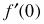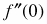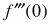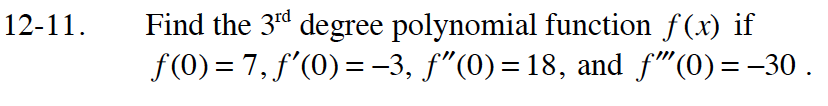### Home > CALC > Chapter 12 > Lesson 12.1.1 > Problem12-11

12-11.

Find the 3rd degree polynomial function f(x) if f(0) = 7,= −3,= 18, and= −30 . Homework Help ✎Use f(0) to solve for d.

Use f(0) to solve for c.